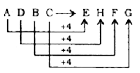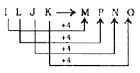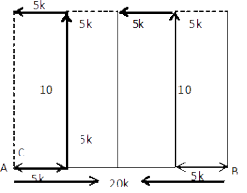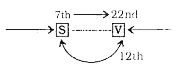# ITC Reasoning Question

DIRECTIONS for questions 1 – 2: In each of the following questions, select the related letters/word/number from the given alternatives.
1. Patrol: Security : : Insurance : ?
1. Money
2. Policy
3. Saving
4. Risk
In order to ensure security, police or defence personnel patrol the area. Similarly, to cover risk, insurance is done.
2. ADBC : EHFG : : ILJK : ?
1. MOPN
2. MPNO
3. ORPQ
4. MPONSimilarly,1. A and B are standing at a distance of 20 km from each other on a straight East-West road. A and B start walking simultaneously eastwards and westwards respectively and both cover a distance of 5 km. Then A turns to his left and walks 10 km. B turns to his right and walks 10 km at the same speed. Then both turn to their left and cover a dis¬tance of 5 km at the same speed. What will be the distance between them?
1. 10km
2. 30km
3. 20km
4. 25kmRequired distance = 5 + 5 = 10 km
2. From the given alternative words, select the word which cannot be formed using the letters of the given word : REFORMATION
1. REFRAIN
2. MOTION
3. REFRACT
4. FORMAT
There is no ‘C’ letter in the keyword so REFRACT cannot be formed.
DIRECTIONS for the question 5: In each of the following questions, select the missing number from the given responses.
3.  169 64 81 30 625 ? 49 50 1296 576 100 70
1. 324
2. 289
3. 441
4. 361
First Row
⇒ √l69+ √64+ √81
= 13 + 8 + 9 = 30
Second Row
⇒ √625 + √? + √49 =50
⇒ 25+ √? +7 = 50
⇒ √? = 50 - 32 = 18
⇒ (?) = (18)2 = 324
Third Row
⇒ √1296 + √576 + √100
= 36 + 24 + 10 = 70
4. Find out a set of numbers amongst the four sets of numbers given in the alternatives, which is the most similar to the numbers given in the question.
Given : (6, 30, 90)
1. 6, 42, 86
2. 7, 42, 218
3. 6, 24, 70
4. 8, 48, 192
6 × 5 = 30, 30 × 3 = 90 8 × 6 = 48, 48 × 4 = 192
5. In a row of boys, Srinath is 7th from the left and Venkat is 12th from the right. If they interchange their positions, Srinath becomes 22nd from the left. How many boys are there in the row?
1. 19
2. 31
3. 33
4. 34Total number of boys in the row = 22 +12 - 1 = 33
DIRECTIONS for questions 8 – 9: In each of the following questions, select the one which is different from the other three responses.
1. Wave
2. Current
3. Tide
4. Strome
Except .Tide, all other terms are related to both air and water. But tide is a regular rise and fall in the level of sea, caused by the attraction of the moon and sun.
1. X
2. Y
3. H
4. D